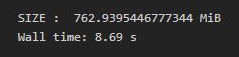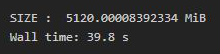# Why is iterating over a dictionary slow in Python?

• Last Updated : 22 Jul, 2021

In this article, we are going to discuss why is iterating over a dict so slow in Python? Before coming to any conclusion lets have a look at the performance difference between NumPy arrays and dictionaries in python:

## Python

 `# import modules ` `import` `numpy as np ` `import` `sys ` ` `  `# compute numpy performance ` `def` `np_performance(): ` `    ``array ``=` `np.empty(``100000000``) ` `    ``for` `i ``in` `range``(``100000000``): ` `        ``array[i] ``=` `i ` `    ``print``(``"SIZE : "``,  ` `          ``sys.getsizeof(array)``/``1024.0``*``*``2``,  ` `          ``"MiB"``) ` ` `  `# compute dictionary performance ` `def` `dict_performance(): ` `    ``dic ``=` `dict``() ` `    ``for` `i ``in` `range``(``100000000``): ` `        ``dic[i] ``=` `i ` `    ``print``(``"SIZE : "``,  ` `          ``sys.getsizeof(dic)``/``1024.0``*``*``2``,  ` `          ``"MiB"``) `

In the above Python Script we have two functions :

1. np_performance: This function creates an empty NumPy array for 10,00,000 elements and iterates over the entire array updating individual element’s value to the iterator location (‘i’ in this case)
2. dict_performance: This function creates an empty Dictionary for 10,00,000 elements and iterates over the entire dictionary updating individual element’s value to the iterator location (‘i’ in this case)

And finally, a sys.getsizeof() function call to calculate the memory usage by respective data structures.

Now we call both these functions, and to measure the time taken by each function we use %time function which gives us the Time execution of a Python statement or expression. %time function can be used both as a line and cell magic:

1. In the inline mode, you can time a single-line statement (though multiple ones can be chained using semicolons).
2. In cell mode, you can time the cell body (a directly following statement raises an error).

Calling these functions from inline mode using %time method :

## Python3

 `# compute time taken ` `%``time np_performance()`

Output:## Python3

 `# compute time taken ` `%``time dict_performance()`

Output:As we can see there is quite a difference in Wall time between iterating on a NumPy array and a python dictionary.

• This difference in performance is due to the internal working differences between arrays and dictionaries as after Python 3.6, Dictionaries in python are based on a hybrid of HashTables and an array of elements. So whenever we take out/delete an entry from the dictionary, rather than deleting a key from that particular location, it allows the next key to be replaced by deleted key’s position. What python dictionary does is replace the value from the hash array with a dummy value representing null. So upon traversing when you encounter these dummy null values it keeps on iterating till it finds the next real-valued key.
• Since there may be lots of empty spaces we’ll be traversing on without any real benefits and hence Dictionaries are generally slower than their array/list counterparts.
• For large-sized Datasets, memory access would be the bottleneck. Dictionaries are mutable, and take up more memory than arrays or (named) tuples (when organized efficiently, not duplicating type information).

My Personal Notes arrow_drop_up
Recommended Articles
Page :